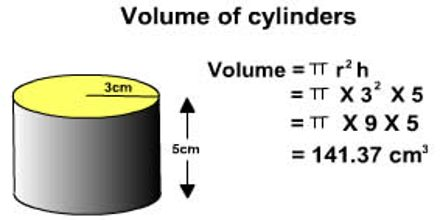Mathematic

# Volume of CylinderThis lecture introduce on Volume of Cylinder with formula and example. The volume of a three-dimensional figure is the amount of space within it. Measured in Units Cubed (e.g. cm³). Here briefly describe on how might we find the Volume of a Cylinder. Since the end (base) of a cylinder is a circle, the area of that circle is given by the formula: area = π r². Multiplying by the height h we get: volume = π r² h; where: π is Pi, approximately 3.142; r is the radius of the circular end of the cylinder and h height of the cylinder.